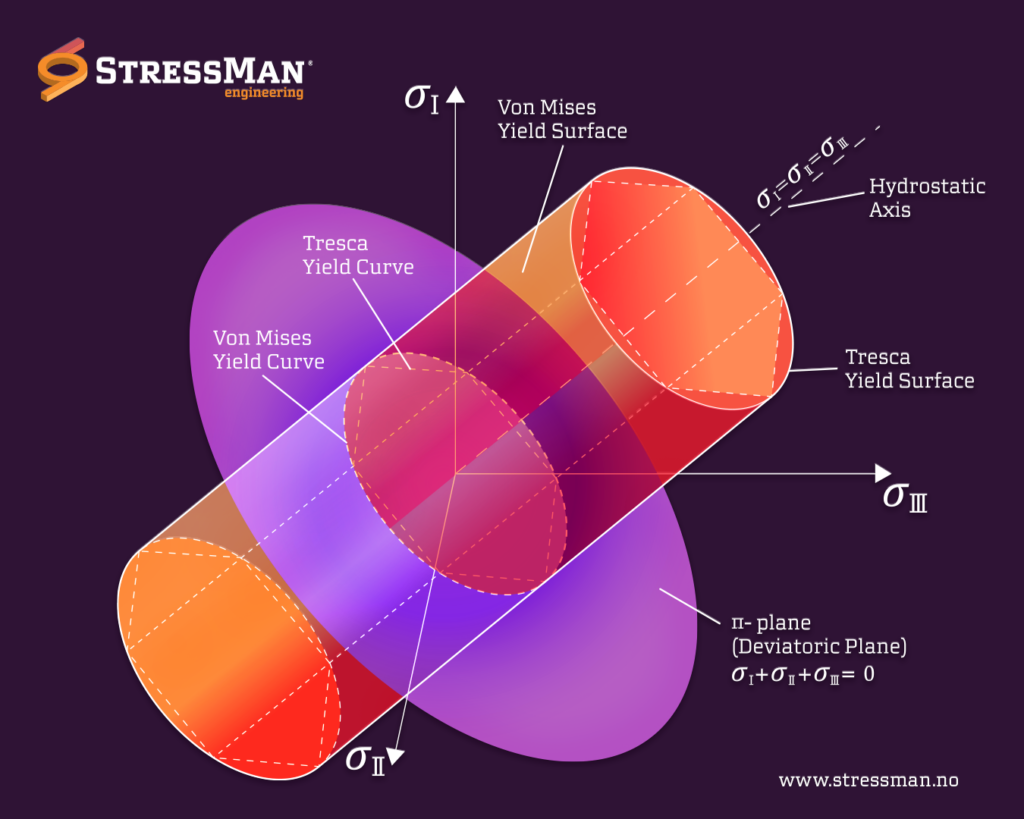## Von Mises stress

Von Mises stress is a critical concept in the field of mechanical engineering that measures the stress state of a material subjected to a complex load. The concept was introduced by the Austrian physicist Richard von Mises in 1913 and is also known as the equivalent stress or von Mises criterion.

In materials science and engineering, stress is defined as the force per unit area that is exerted on a material. When a material is subjected to an external force, it experiences stress, which can lead to deformation or failure if it exceeds the material’s strength. Stress can be categorized into three types: compressive stress, tensile stress, and shear stress.

When a material is subjected to a complex load, the stress state cannot be determined by a single stress component. Instead, it is determined by the combination of three principal stresses acting on the material in three different directions. The von Mises stress is a scalar quantity that represents the equivalent stress produced by the combination of the principal stresses.

The von Mises stress is a useful measure for predicting when a material will yield or deform permanently. It is commonly used in the design and analysis of mechanical components and structures to ensure that they can withstand the expected loads without failure.

The von Mises stress is calculated as the square root of the sum of the squared differences between the three principal stresses. It is expressed mathematically as:

σvM = √(σ1 – σ2)^2 + (σ2 – σ3)^2 + (σ3 – σ1)^2

where σ1, σ2, and σ3 are the principal stresses.

In summary, von Mises stress is an essential concept in materials science and engineering that provides a measure of the equivalent stress state in a material subjected to a complex load. By using the von Mises stress, engineers can accurately predict the material’s behavior and design components that can withstand the expected loads.